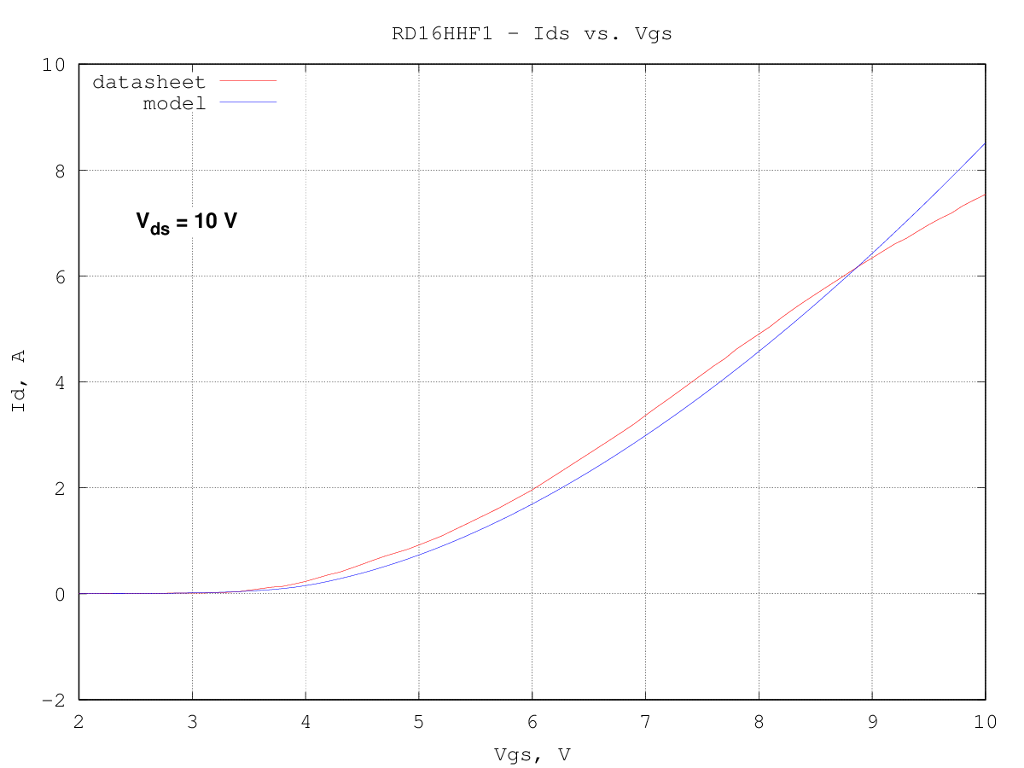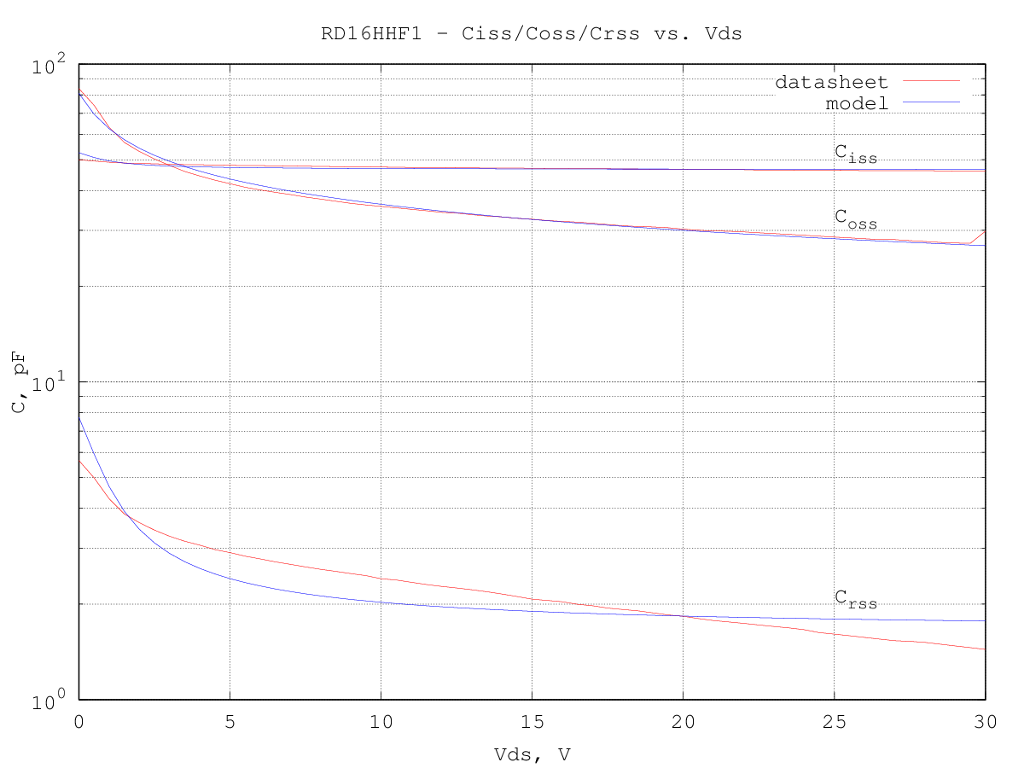# Mitsubishi RD16HHF1 LDMOS LTspice model

Under construction...

The device has been modeled using the LTspice VDMOS model, since it is well suited also for LDMOS devices and contains only few of parameters which can be guessed from the scarce data available from the datasheet.
The static (drain current) and dynamic (capacitances) characteristics were separately optimized, starting from a first guess extracted from the curves in the datasheet. The optimized model description is:

.model RD16HHF1 VDMOS(Rg=987m Rd=0m Rs=111m Vto=3.19 Kp=0.495 Lambda=0 mtriode=0.804 subthres=2.37e-02 Cgdmax=11.6p Cgdmin=1.64p Cgs=44.9p Cjo=73.1p M=0.297 Vj=0.839)

the quality of the fit is not exceptional, which is to be expected since the device model used (VDMOS) is more targeted toward modeling the switching characteristics of power mosfets, but should be good enough for a first design pass.

Here below is a graph of the drain current vs. the drain voltages for several values of the gate voltage; fit is relatively good at medium currents, but a bit off in the linear region:The Ids vs. Vgs curve fit, for a fixed drain voltage of 10 V, is relatively good:Input (Ciss) and output (Coss) capacitances are well modeled, while the reverse transfer capacitance (Crss) is quite off, as the voltage dependency is not matching with the one implemented in the LTspice model: